# Power Factor – Importance, Calculation and Correction techniques

In an AC power system, the power factor is a very important parameter that defines how efficiently electrical power is being utilized by the load. It is a rational number between -1 and 1 but has no unit. The p.f of a system depends on the type of load present, whether resistive, inductive or capacitive. The inductive and capacitive load has a negative impact on the p.f. of the system.  Poor p.f results in an increase in current drained by the load.

## Definition of power factor

Power factor can be defined as the ratio of real power (Active power) to the apparent power. It can also be defined as the absolute value of the cosine of the phase shift between the voltage and current in an AC circuit. It is denoted by the Greek alphabet λ (Lambda).

Power factor (λ) = Active power/Apparent power
= VI.COS φ/ VI
= COS φ

‘V’ is voltages in Volts
‘I’ is current in Amperes
‘Φ’ is the phase angle between voltage and current

## Active power (kW)

It is the true power transmitted to the load for energy conversion. For example, motor consumes the true power from the circuit and convert it into mechanical power, whereas lamps, on the other hand, convert the same into light. It is represented by the letter P.

## Reactive power (kW)

Reactive power is the power required to produce the magnetic field in motors and transformer and has a direct impact on the p.f. It is represented by the letter Q.

## Apparent power (kVA)

Apparent power is the product of voltage and current consumed by a load irrespective of their phase angle. It is the combination of real and reactive powers. It is represented by the letter S.

## Unity power factor

Unity power factor is considered as a perfect scenario, during which apparent power and the real power shall be in phase. When the load is purely resistive, the current flow to the load will be linear and hence the phase shift between the voltage and current will be zero and cos Φ will be unity.

Power factor is considered to be leading if the apparent power leads the real power (true power), (i.e.) the current leads voltage. Capacitive loads cause the current to lead the voltage so as the power factor.

## Lagging power factor

Power factor is considered to be leading if the apparent power lags the real power (true power), (i.e.) the current lags voltage. Inductive loads cause the current to lag the voltage so as the p.f.

## Power factor Calculation

From the power triangle:
Power factor = Real power/Apparent power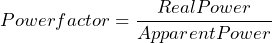Also,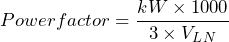Also,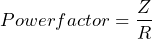## Why is power factor improvement important?

Power factor improvement aims at optimal utilization of electrical power, reduction of electricity bills and reduction of power loss.

• Power transformers are independent of P.F. If the power factor is close to unity, for the same KVA rating of the transformer more load can be connected. (Better the power factor lesser will be the current flow).
• Penalties imposed by the power utility companies for non-maintenance of optimal p.f. can be avoided.
• Optimal sizing of power cables is possible if the power factor. Low p.f. results in higher copper loss (I2R) loss also more voltage shall be dropped across the cable.

## Power factor correction techniques

Most of the power loads are inductive and causes the current to lag the voltage. In order to overcome this few power factor correction techniques are adapted, that helps in neutralizing this lagging current. The most common P.F. correction technique is the usage of static capacitors in parallel to the load. Static capacitors supply leading current to the system and reduce the lag. Capacitor banks are connected in parallel to inductive loads. These capacitors are switched using a contactor based on the requirement. Static VAR compensators are also used for p.f. correction. These are power electronic version of reactive power compensators and make use of thyristors for capacitor switching instead of contactors.

Other power factor correction techniques include connecting synchronous compensators parallel to the load. They are synchronous motors run at no load. When a synchronous motor is overexcited and run at no load it acts as a capacitor and supplies reactive power to the network. Synchronous compensators are connected in parallel to the load.

## Power factor correction calculation

Appropriate power factor corrective measure has to be taken for maintaining the required power factor of the system. Most of the times, engineers opt for capacitor banks for p.f. correction. Here is how the capacitor required for p.f. correction is determined:

We can measure the supply voltage using a voltmeter and the current drawn by the load using an ammeter. From these data we can calculate the current p.f., apparent power and reactive power consumed by the load, using the formulae below.

Apparent power = V x I (Measured using ammeter and voltmeter)
Current power factor = Load KW (Real power) / Apparent power

From the power triangle:

Reactive power (kVAR) = Sq.rt((Apparent power-kVA)2 – (Real power-kW)2)

And,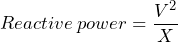From the above equation,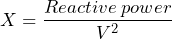Calculating the size of the capacitor used for achieving unity power factor can be calculated as follows: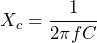Hence,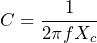Where,

C is the value of capacitance in Farads

F is the supply frequency

Xc is the capacitive reactance.

## Importance of Power Factor/ Significance of power factor.

Active power (True power) is expressed as:

P= VI.Cos Φ

For a given load P shall be always constant and voltage supplied by the source V shall also be constant. The parameters I and Cos Φ are interdependent. For instance, if the value of Cos Φ is unity, then the current drained by the load from the source shall be: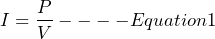And if the p.f. Cos Φ is less than unity, say ‘0.8’, then the current drained by the load from the source shall be: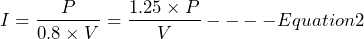From the expressions 1 and 2 it is clear that while transmitting the same amount of power P at lesser p.f., the current has increased significantly. Hence, for a constant load, at a constant voltage, the current drained from the source is inversely proportional to the power factor.

Increase in current directly impacts the cost of power generation and also increases transmission losses. The conductor used in an equipment is designed to pass a particular amount of current through it. If the power factor of the supply is poor, more current may flow to the equipment and can either damage the equipment or reduce the life expectancy.

Utilities impose huge penalties on commercial consumers who have a p.f. below a certain level. Therefore, it is very important to maintain the power factor at a certain value for the effective utilization of power.